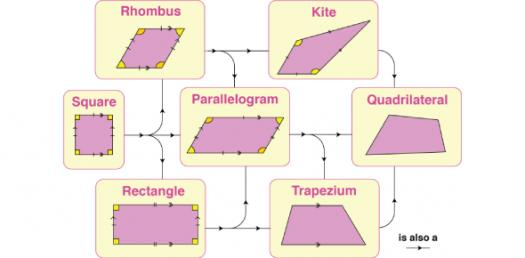# Ultimate Trivia Test On Quadrilaterals! Geometry Quiz

25 Questions | Total Attempts: 29Settings.

Related Topics
• 1.
What is x in the parallelogram below?
• A.

22

• B.

64

• C.

11

• D.

3

• 2.
In the parallelogram below, what is x?
• A.

139

• B.

119

• C.

34.75

• D.

20.25

• 3.
What is the measure of angel 1 in the parallelogram below?
• A.

54°

• B.

126°

• C.

36°

• D.

180°

• 4.
Which of the following are true about rectangles?I. Their opposite sides are congruent.II. They have four right angles.III. Their diagonals are congruent.
• A.

I only

• B.

II only

• C.

III only

• D.

I and II only

• E.

I, II and III

• 5.
What is the measure of angle D?
• A.

65°

• B.

115°

• C.

90°

• D.

25°

• 6.
What is the name of the quadrilateral in the diagram?
• A.

Trapezoid

• B.

Rectangle

• C.

Rhombus

• D.

Parallelogram

• 7.
Find the values of x and y in the diagram giving reasons for your answer.
• 8.
What is x in the parallelogram below?
• A.

90

• B.

6

• C.

22

• D.

12

• 9.
If angle C=65 an angle E = 5X, what is x?
• A.

13°

• B.

25°

• C.

65°

• D.

90°

• 10.
In the rectangle ABCD below, AB = 4, BC = 8. Find the length of AD.
• 11.
If AE = 5, what is CE?
• 12.
If DE = 7, what is BD?
• 13.
In the rectangle below, AE = 4x + 3 and CE = 2x + 5. Find the length of AC.Remember to solve for x and then plug it back in to the expressions for AE and CE.
• 14.
Given parallelogram ABCD, find the measure of angle A if angle B = 130 degrees.
• 15.
In parallelogram ABCD, angle B = 3x + 20 degrees and angle D = 4x - 10 degrees. Find x.
• 16.
In parallelogram ABCD, AB = 3x + 4 and CD = 12x - 5. Find the length of CD.
• 17.
In parallelogram ABCD, angle A = 2x + 20 degrees and angle B = 4x + 10 degrees.Find the measure of angle A.
• 18.
In parallelogram ABCD, diagonal AC = 16 and AE = 4x. Find x.
• 19.
In parallelogram ABCD, BE = 5x - 2 and DE = 3x + 8. Find the length of BD.
• 20.
What is the measure of angle J in the parallelogram below?
• A.

22

• B.

64

• C.

87

• D.

93

• 21.
Quadrilateral ABCD is a rectangle.If AG = 2j + 21 and DG = - j + 39, find BD.
• A.

33

• B.

6

• C.

66

• D.

16.5

• 22.
Quadrilateral ABCD is a rectangle.If angle ADB = 8k + 10 and angle CDB = 9k - 56, find  angle CBD.
• A.

8

• B.

45

• C.

74

• D.

16

• 23.
• A.

RT = 2.68

• B.

RT = 3.0

• C.

RT = 7.4

• D.

RS = 3.0

• E.

RS = 8.4

• F.

RS = 7.4

• G.

Angle T = 3.0

• H.

Angle T = 58

• I.

Angle T = 68

• 24.
• A.

HI = 31

• B.

HI = 6

• C.

HI = 7.4

• D.

GH = 3.6

• E.

GH = 8.4

• F.

GH = 7.4

• G.

Angle I = 6

• H.

Angle I = 31

• I.

Angle I = 68